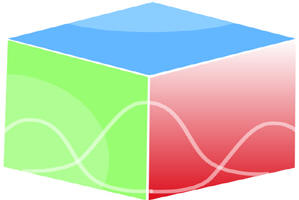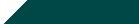``Home| Journals | Statistics Online Expert | About Us | Contact UsUntitled Document

[

A Note on the Finite Sample Properties of Ridge Estimator

{1Professor and HOD, 2, 3Research Scholars}, Department of Statistics, M. D. University, Rohtak, Hariyana, INDIA.

Research Article

Abstract: The article studies the finite sample properties of generalized ridge regression estimator using a different approach. A comparative study of relative bias and relative efficiency of the estimator with respect to the ordinary least square have been made empirically. The results have also been compared with the existing results and are found to be quite different from those already existing in literature.

Keywords: Ordinary Least Squares, Generalized Ridge Regression (GRR), Relative Bias, Relative MSE, Relative Efficiency, Finite Sample Properties.

Introduction

In linear regression models, Ridge Regression is perhaps the most widely used technique in the presence of multicollinearity. Proposed by Hoerl and Kennard ,  the Ridge Regression Estimator is characterized by a scalar, the choice of which is subjective requiring the judgment of the analyst. However, working with the canonical form of the regression model Hoerl and Kennard  defined general ridge regression estimator suggesting an initial choice of the characterizing scalar. Extensive work has been carried out since then, a good account of which is available in Vinod and Ullah  and . Working with the initial choice of the characterizing scalar, Dwivedi et al.  worked out the first two moments of individual coefficients of GRR estimator assuming error distribution to be normal. Hemmerle and Carey also worked out exact properties of two different forms of GRR estimators but demonstrated that the one suggested by Hoerl and Kennard  performs better in terms of relative bias and relative risk. It will not be out of context to mention that the estimators perform differently when sample size is small and it is more so in the presence of multicollinearity as negative effects of multicollinearity are magnified in smaller samples. Owing to this, assuming error distribution to be normal the paper attempts to assess the finite sample behavior of generalized ridge regression. For this purpose the relative bias, relative mean squared error and the relative efficiency of the estimator in comparison to OLS have been evaluated numerically and compared with the existing results. Interestingly, the expressions of relative bias and relative risk are found to be different from the existing results obtained by Dwivedi et al. . The following section describes the estimator and its properties and empirically enumerates the results. A brief outline of proof of the theorem is provided in the end.

The Estimator and its Properties

Consider the canonical form of the linear regression model

(2.1)

where y is an vector of  observation on the dependent variables, X is an  full column rank matrix of  observation of  explanatory variables, and  vector of unknown regression coefficients . The elements of disturbance vector u are assumed to be i.i.d each following normal distribution with mean zero and variance σ2 so that

(2.2)

Following , we can write

This canonical reduction can be obtained by using singular value decomposition of the  matrix X (see, , p5-6). Using it the general ridge regression estimator is given by

(2.3)

and K is a diagonal matrix with nonnegative elements k1,k2,…kp as the characterizing scalar, and

is the ordinary least square estimator of . Clearly, GRR is a biased estimator, with bias vector

(2.4)

and

(2.5)

as  and K are assumed to be diagonal matrices,  is also diagonal and

(2.6)

Provided  ‘s are non-stochastic.

Now minimizing the expression (2.6) term by term i.e. minimizing the diagonal elements of the mean squared error matrix of (2.5) with respect to  yields

(2.7)

(2.8)

where  is the ith element of the ordinary least squares estimator b of  and

(2.9)

is an unbiased estimator of  where

(2.10)

where the i th element of is given by

(2.11)

For finite sample sizes, the following theorem gives the first and second moments of

Theorem: Assuming normality of errors the first and second moments of of (2.11) are given by

(2.12)

(2.13)

where  is the non-centrality parameter. Using (2.12) and (2.13) we can compute the bias and mean squared error of  .

Proof: see Appendix.

Using these, it is easy to compute the relative bias and relative mean squared error using

(2.14)

and

(2.15)

Respectively. The efficiency of the OLS relative to GRR estimator is obtained from

(2.16)

The values of  and have been tabulated for a few selected vales of  and .These results are provided in Tables 2.1, 2.2, 2.3 respectively and have been graphed for selected values of  and.

The expressions (2.12) and (2.13) are clearly different from those obtained by Dwivedi et al.  and therefore a substantial difference is observed numerically. Unlike the results obtained by Dwivedi et al. , the magnitude of relative bias is found to be decreasing function of the non-centrality parameter and an increasing function of degrees of freedom, so long as  and . However, for  the magnitude of relative bias is found to be increasing. Interestingly, as  increases the relative bias tends to -1, justification of this easily comes from the fact that the Ridge regression estimator shrinks the parameter vector towards zero. The relative MSE and relative efficiency are also observed to be decreasing for specific values of  and Hence, the finite sample properties of the Ridge regression estimator are not only heavily dependent upon the non-centrality parameter but on the degrees of freedom as well. It is also pertinent to mention that ambiguity in the numerical computations in relative bias, relative MSE and relative efficiency are found in the paper by Dwivedi et al.  when  which are evident in the respective tables.

Table 2.1: Relative Bias for specific values of non-centrality parameter and degrees of freedom

 1 2 5 10 20 50 0.01 E0 -0.24917 -0.28795 -0.46717 -0.73385 -0.9078 -0.98448 E1 -0.249 -0.287 -0.318 -0.33 -0.337 -0.353 0.05 E0 -0.24588 -0.28419 -0.47043 -0.73901 -0.91041 -0.98502 E1 -0.246 -0.283 -0.313 -0.325 -0.332 -0.348 0.1 E0 -0.24187 -0.27962 -0.47464 -0.74536 -0.91358 -0.98566 E1 -0.242 -0.278 -0.307 -0.319 -0.326 -0.341 0.5 E0 -0.21306 -0.24776 -0.5126 -0.79201 -0.93533 -0.98992 E1 -0.213 -0.243 -0.268 -0.278 -0.283 -0.296 0.7 E0 -0.2006 -0.2347 -0.53362 -0.81263 -0.94412 -0.99155 E1 -0.201 -0.228 -0.251 -0.26 -0.265 -0.276 0.9 E0 -0.18924 -0.22337 -0.55544 -0.83154 -0.95175 -0.99292 E1 -0.189 -0.215 -0.236 -0.244 -0.248 -0.258 1 E0 -0.18394 -0.21832 -0.56653 -0.84038 -0.95518 -0.99352 E1 -0.184 -0.208 -0.228 -0.236 -0.241 -0.25 2 E0 -0.14196 -0.18716 -0.67665 -0.90889 -0.97873 -0.99732 E1 -0.142 -0.158 -0.172 -0.177 -0.18 -0.185 5 E0 -0.1107 -0.25493 -0.89913 -0.98566 -0.99789 -0.99982 E1 -0.08 -0.086 -0.091 -0.093 -0.094 -0.095 10 E0 -0.56774 -0.70662 -0.99125 -0.99952 -0.99996 -1 E1 -0.045 -0.047 -0.048 -0.049 -0.049 -0.049 50 E0 -1 -1 -1 -1 -1 -1 E1 -0.014 -0.014 -0.014 -0.014 -0.014 -0.014

E0 –Results by our approach

E1-Results by Dwivedi et al. .

Table 2.2: Relative MSE for specific values of non-centrality parameter and degrees of freedom

 1 2 5 10 20 50 0.01 E0 31.5301 28.3576 19.3043 8.59851 2.94502 1.17679 E1 31.53 28.423 28.85 24.837 24.267 22.754 0.05 E0 6.52534 5.92247 4.07935 2.14614 1.24542 1.010629 E1 6.525 5.94 5.454 5.263 5.155 4.87 0.1 E0 3.3946 3.11178 2.17857 1.34888 1.03842 0.991026 E1 3.395 3.123 2.898 2.809 2.758 2.626 0.5 E0 0.853875 0.819369 0.686614 0.779676 0.909809 0.982917 E1 0.854 0.827 0.804 0.795 0.789 0.776 0.7 E0 0.657999 0.63841 0.598543 0.768053 0.914648 0.985032 E1 0.658 0.646 0.635 0.631 0.628 0.622 0.9 E0 0.543213 0.530987 0.561328 0.774361 0.922711 0.987153 E1 0.543 0.538 0.533 0.531 0.53 0.527 1 E0 0.501285 0.570042 0.552523 0.780415 0.927023 0.988142 E1 0.501 0.498 0.495 0.494 0.493 0.492 2 E0 0.289568 0.292192 0.593021 0.858859 0.962787 0.994925 E1 0.29 0.292 0.294 0.295 0.295 0.296 5 E0 0.118409 0.213709 0.854008 0.975889 0.996133 0.999642 E1 0.123 0.123 0.124 0.124 0.124 0.124 10 E0 0.455941 0.620464 0.986397 0.999155 0.999928 0.999996 E1 0.059 0.058 0.058 0.058 0.057 0.057 50 E0 1 1 1 1 1 1 E1 0.013 0.013 0.013 0.013 0.013 0.013

E0 –Results by our approach

E1-Results by Dwivedi et al. .

Graph: Showing relative bias and relative MSE of GRR Estimator (Figure 1 and Figure2)

Table 2.3: Relative Efficiency for specific values of non-centrality parameter and degrees of freedom

 1 2 5 10 20 50 0.01 E0 63.0601 56.7153 38.6087 17.197 5.89003 2.35358 E1 63.06 56.846 51.701 49.674 48.534 45.508 0.05 E0 65.2534 59.2247 40.7935 21.4614 12.4542 10.10629 E1 65.253 59.398 54.542 52.626 51.548 48.698 0.1 E0 67.8919 62.2356 43.5713 26.9776 20.7684 19.82052 E1 67.892 62.465 57.955 56.17 55.167 52.527 0.5 E0 85.3875 81.9369 68.6614 77.9676 90.9809 98.29169 E1 85.388 82.69 80.394 79.46 78.937 77.632 0.7 E0 92.1198 89.3774 83.796 107.527 128.051 137.9045 E1 92.12 90.405 88.907 88.282 87.936 87.106 0.9 E0 97.7784 95.5776 101.039 139.385 166.088 177.6875 E1 97.779 96.845 95.985 95.607 95.403 94.949 1 E0 100.257 114.008 110.504 156.083 185.405 197.6283 E1 100.258 99.65 99.056 98.783 98.638 98.34 2 E0 115.827 116.877 237.208 343.543 385.115 397.9702 E1 115.883 116.927 117.683 117.94 118.099 118.569 5 E0 118.409 213.709 854.008 975.889 996.133 999.6423 E1 123.245 123.494 123.602 123.635 123.653 123.833 10 E0 911.883 1240.93 1972.79 1998.31 1999.86 1999.992 E1 117.495 116.319 115.394 115.026 114.891 114.656 50 E0 10000 10000 10000 10000 10000 10000 E1 129.538 128.682 128.197 128.039 127.988 127.827

E0 –Results by our approach

E1-Results by Dwivedi et al. .

Appendix

In order to find the expression (2.12) of the theorem, let us define

(A.1)

where xi (i=1,2…p) is the ith column vector of X. Since is the OLS estimator of  following

N(), therefore, the distribution of  where  .

Next, the distribution of is  with  degrees of freedom and is independent of the distribution of. Using, we can write

(A.2)

(A.3)

(A.4)

Following  we can write the above equation as

We notice that the value of integral is zero for odd values of j because then the power of z is odd. Dropping such terms we have

(A.5)

Using the duplication formula above expression becomes

(A.6)

The integral part

is computed using the transformations

which gives the integral part as

(A.7)

Substituting the above value of (A.7) and using it in (A.6) we get the first raw moment of as given in the theorem.

Proceeding in the same way the second and higher raw moments of  can be obtained.

References

1. Dwivedi, T. D. Srivastava, V. K. Hall, R. L., “Finite Sample Properties of Ridge Estimators”. Technometrics, Vol. 22, No. 2. Pp205-212, 1980.
2. Hemmerle, W. J. and Carey, M.B., "Some Properties of Generalized Ridge estimators" Communications in Statistics: Simulation and Computation. 12:3, 239-253, 1983.
3. Hoerl, A. E. and Kennard, R. W., “Ridge Regression Biased Estimation for Non-Orthogonal Problems”. Technometrics, 12, 55-67, 1970a.
4. Hoerl, A. E. and Kennard, R. W. “Ridge Regression Applications to Non-Orthogonal Problems”. Technometrics, 12, 69-82, 1970b.
5. Judge, G.G. and Bock, M.E., the Statistical Implications of Pre-Test and Stein-Rule Estimators in Econometrics. North Holland Publishing Company, Amsterdam, 1978.
6. Marvin, H.J. Gruber, Regression Estimators: A Comparative Study. The John Hopkins University Press, 2010.
7. Vinod, H.D. and Ullah, A., Recent Advance in Regression Analysis. Marcel Dekker, New York, 1981.